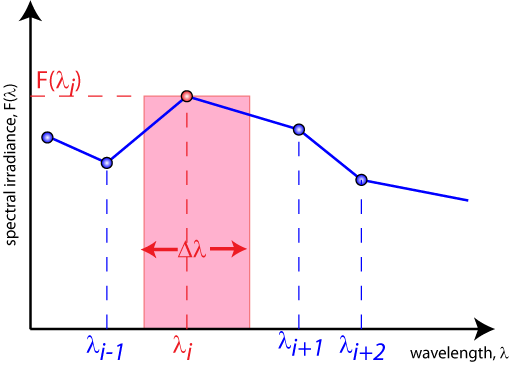The total power density emitted from a light source can be calculated by integrating the spectral irradiance over all wavelengths or energies

$H={\int }_{0}^{\infty }F\left(\lambda \right)d\lambda$

where:

H is the total power density emitted from the light source in W m-2;
F(λ) is the spectral irradiance in units of Wm-2μm-1; and
is the wavelength.

However, a closed form equation for the spectral irradiance for a light source often does not exist. Instead the measured spectral irradiance must be multiplied by a wavelength range over which it was measured, and then calculated over all wavelengths. The following equation can be used to calculate the total power density emitted from a light source.

$H=\sum _{i}F\left(\lambda \right)\text{Δ}\lambda$

H is the total power density emitted from the light source in W m-2;
F(λ) is the spectral irradiance in units of Wm-2μm-1; and
Δλ is the wavelength.Calculating the total power density from a source requires integrating over the spectrum by calculating the area of each element and then summing them together.

Measured spectra are typically not smooth as they contain emission and absorption lines. The wavelength spacing is usually not uniform to allow for more data points in the rapidly changing parts of the spectrum. The spectral width is calculated from the mid-points between two the adjacent wavelengths.

$\begin{array}{c}\Delta \lambda =\frac{{\lambda }_{i+1}+{\lambda }_{i}}{2}-\frac{{\lambda }_{i}+{\lambda }_{i-1}}{2}\\ =\frac{{\lambda }_{i+1}-{\lambda }_{i-1}}{2}\end{array}$

Power in each segment is then:

${H}_{i}=\Delta \lambda \cdot F\left({\lambda }_{i}\right)$

Summing all the segments gives the total power H as in the equation above.